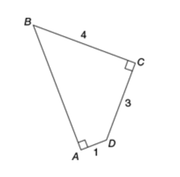• 7 CATs FREE!
If you earn 100 Forum Points

Engage in the Beat The GMAT forums to earn
100 points for \$49 worth of Veritas practice GMATs FREEVERITAS PRACTICE GMAT EXAMS
Earn 10 Points Per Post
Earn 10 Points Per Thanks
Earn 10 Points Per Upvote

## In quadrilateral ABCD above, what is the length of AB ?

##### This topic has expert replies
Master | Next Rank: 500 Posts
Posts: 394
Joined: 02 Jul 2017
Thanked: 1 times
Followed by:4 members

### In quadrilateral ABCD above, what is the length of AB ?

by AbeNeedsAnswers » Fri May 03, 2019 6:02 pm

00:00

A

B

C

D

E

## Global StatsIn quadrilateral ABCD above, what is the length of AB ?

A) âˆš26
B) 2âˆš5
C) 2âˆš6
D) 3âˆš2
E) 3âˆš3

C

Source: Official Guide 2020

### GMAT/MBA Expert

GMAT Instructor
Posts: 14498
Joined: 08 Dec 2008
Location: Vancouver, BC
Thanked: 5254 times
Followed by:1262 members
GMAT Score:770
by Brent@GMATPrepNow » Sat May 04, 2019 6:00 am
AbeNeedsAnswers wrote:In quadrilateral ABCD above, what is the length of AB ?

A) âˆš26
B) 2âˆš5
C) 2âˆš6
D) 3âˆš2
E) 3âˆš3

C

Source: Official Guide 2020
[spoiler=]
2019-04-26_1235.png
[/spoiler][/quote]
First add a line to join B and D:If we focus on the blue right triangle, we can EITHER recognize that legs of length 3 and 4 are part of the 3-4-5 Pythagorean triplet, OR we can apply the Pythagorean Theorem.Either way, we'll see that the triangle's hypotenuse (BD) must have length 5

Now, when we focus on the red right triangle, we can . . .. . . apply the Pythagorean Theorem to write: xÂ² + 1Â² = 5Â²
Simplify: xÂ² + 1 = 25
So: xÂ² = 24
So: x = âˆš24 = âˆš[(4)(6)] = (âˆš4)(âˆš6) = 2âˆš6

Cheers,
Brent
Brent Hanneson - Creator of GMATPrepNow.com
Use my video course along with Beat The GMAT's free 60-Day Study GuideWatch these video reviews of my course
And check out these free resources

Legendary Member
Posts: 1496
Joined: 29 Oct 2017
Followed by:5 members
by swerve » Sat May 04, 2019 10:44 am
If we draw diagonal $$BD$$, we've created two right triangles: $$BCD$$ and $$BAD$$. We see that triangle $$BCD$$ is a $$3-4-5$$ right triangle. So we see that side $$BD = 5$$.

Therefore, triangle $$BAD$$ is a right triangle with a leg of $$1$$ and a hypotenuse of $$5$$. We can let side $$AB = n$$ and use the Pythagorean theorem to determine $$n$$.
\begin{align*} 1^2 + n^2 &= 5^2\\ 1 + n^2 &= 25\\ n^2 &= 24\\ n &= \sqrt{24}\\ &= \sqrt{4} \cdot \sqrt{6} \\ &= 2\sqrt{6} \end{align*}

### GMAT/MBA Expert

Elite Legendary Member
Posts: 10346
Joined: 23 Jun 2013
Location: Palo Alto, CA
Thanked: 2867 times
Followed by:503 members
GMAT Score:800
by Rich.C@EMPOWERgmat.com » Wed May 22, 2019 8:48 am
Hi All,

When dealing with 'weird' shapes, it often helps to break the shape down into 'pieces' that are easier to deal with. Here, if you draw a line from B to D, you will from 2 RIGHT TRIANGLES.

Triangle BCD has legs of 3 and 4, so it's a 3/4/5 right triangle.
Triangle BAD then has a leg of 1 and a hypotenuse of 5. We can use the Pythagorean Formula to find the missing leg...

1^2 + B^2 = 5^2
1 + B^2 = 25
B^2 = 24

From here, if we square-root both sides, we'll have...
B = âˆš24
B = 2âˆš6